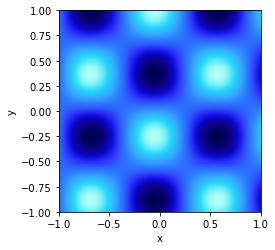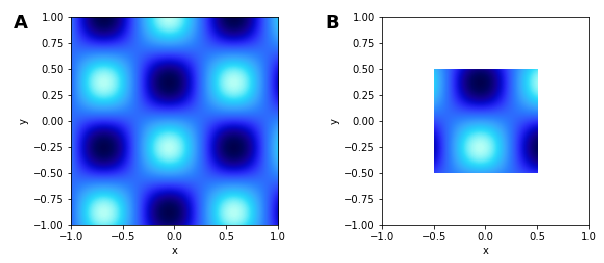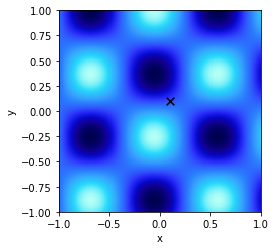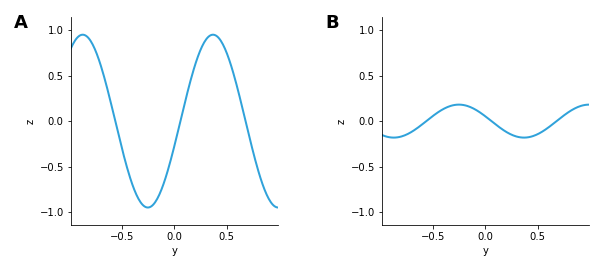# Image#

Title
Image Element
Dependencies
Matplotlib
Backends
Matplotlib
Bokeh
Plotly
```import numpy as np
import holoviews as hv
hv.extension('matplotlib')
```Image represents a regularly sampled 2D grid of an underlying continuous space of intensity values, which will be colormapped on plotting. The grid of intensity values may be specified as a `NxM` sized array of values along with a bounds, but it may also be defined through explicit and regularly spaced x/y-coordinate arrays of shape `M` and `N` respectively. The two most basic supported constructors of an Image therefore include:

```Image((X, Y, Z))
```

where `X` is a 1D array of shape `M`, `Y` is a 1D array of shape `N` and `Z` is a 2D array of shape `NxM`, or equivalently:

```Image(Z, bounds=(x0, y0, x1, y1))
```

where `Z` is a 2D array of shape `NxM` defining the intensity values and the bounds define the (left, bottom, right, top) edges of four corners of the grid. Other gridded formats which support declaring of explicit x/y-coordinate arrays such as xarray are also supported. See the Gridded Datasets user guide for all the other accepted data formats.

```ls = np.linspace(0, 10, 200)
xx, yy = np.meshgrid(ls, ls)

bounds=(-1,-1,1,1)   # Coordinate system: (left, bottom, right, top)
img = hv.Image(np.sin(xx)*np.cos(yy), bounds=bounds)
img
```Slicing, sampling, etc. on an `Image` all operate in this continuous space, whereas the corresponding operations on a `Raster` work on the raw array coordinates.

```img + img[-0.5:0.5, -0.5:0.5]
```Notice how, because our declared coordinate system is continuous, we can slice with any floating-point value we choose. The appropriate range of the samples in the input numpy array will always be displayed, whether or not there are samples at those specific floating-point values. This also allows us to index by a floating value, since the `Image` is defined as a continuous space it will snap to the closest coordinate, to inspect the closest coordinate we can use the `closest` method:

```closest = img.closest((0.1,0.1))
points = hv.Points([closest])

print('The value at position %s is %s' % (closest, img[0.1, 0.1]))

img * points.opts(color='black', marker='x', s=60)
```
```The value at position (0.105, 0.095) is 0.1293472017023185
```We can also easily take cross-sections of the Image by using the sample method or collapse a dimension using the `reduce` method:

```img.sample(x=0) + img.reduce(x=np.mean)
```The constructor of `Image` attempts to validate the input data by ensuring it is regularly sampled. In some cases, your data may be not be regularly sampled to a sufficiently high precision in which case you qill see an exception recommending the use of `QuadMesh` instead. If you see this message and are sure that the `Image` element is appropriate, you can set the `rtol` value in the constructor to allow a higher deviation in sample spacing than the default of `10e-6`. Alternatively, you can set this globally using `hv.config.image_rtol` as described in the Installing and Configuring user guide.

One additional way to create Image objects is via the separate ImaGen library, which creates parameterized streams of images for experiments, simulations, or machine-learning applications.

For full documentation and the available style and plot options, use `hv.help(hv.Image).`

This web page was generated from a Jupyter notebook and not all interactivity will work on this website. Right click to download and run locally for full Python-backed interactivity.